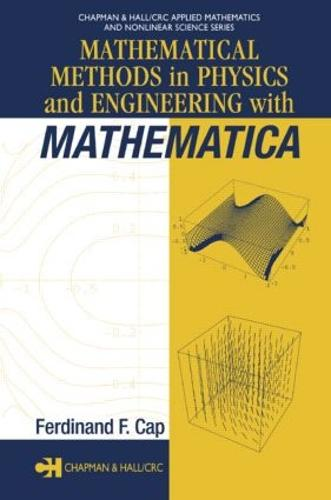•Mathematical Methods in Physics and Engineering with Mathematica - Chapman & Hall/CRC Applied Mathematics & Nonlinear Science (Hardback)

(author)
£140.00
Hardback 352 Pages / Published: 28/05/2003
• We can order this

Usually dispatched within 3 weeks

More than ever before, complicated mathematical procedures are integral to the success and advancement of technology, engineering, and even industrial production. Knowledge of and experience with these procedures is therefore vital to present and future scientists, engineers and technologists.

Mathematical Methods in Physics and Engineering with Mathematica clearly demonstrates how to solve difficult practical problems involving ordinary and partial differential equations and boundary value problems using the software package Mathematica (4.x). Avoiding mathematical theorems and numerical methods-and requiring no prior experience with the software-the author helps readers learn by doing with step-by-step recipes useful in both new and classical applications.

Mathematica and FORTRAN codes used in the book's examples and exercises are available for download from the Internet. The author's clear explanation of each Mathematica command along with a wealth of examples and exercises make Mathematical Methods in Physics and Engineering with Mathematica an outstanding choice both as a reference for practical problem solving and as a quick-start guide to using a leading mathematics software package.

Publisher: Taylor & Francis Inc
ISBN: 9781584884026
Number of pages: 352
Weight: 612 g
Dimensions: 235 x 159 x 20 mm